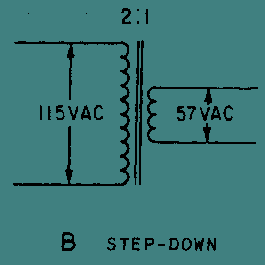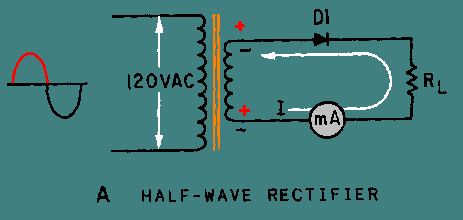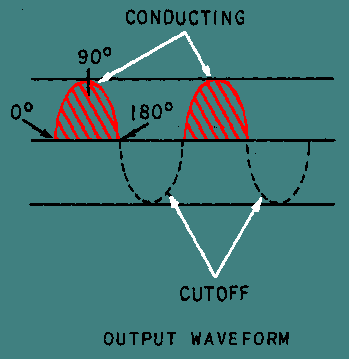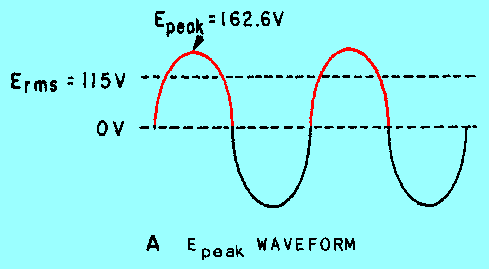power supply is completely insulated, it presents a dangerous shock hazard to anyone who comes in contact with it. When a transformer is not being used, the return side of the ac line is connected to the metal chassis.">Custom SearchIn some cases a power supply may not use a transformer; therefore, the power supply would be connected directly to the source line voltage. This type of connection is used primarily because it is economical. However, unless the power supply is completely insulated, it presents a dangerous shock hazard to anyone who comes in contact with it. When a transformer is not being used, the return side of the ac line is connected to the metal chassis. To remove this potential shock hazard and to have the option of stepping up or stepping down the input voltage to the rectifier, a transformer must be used.

View A of figure 4-2 shows the schematic diagram for a STEP-UP transformer; view B shows a STEP-DOWN transformer; and, view C shows a STEP-UP, CENTER-TAPPED transformer. The step-up and step-down transformers were discussed in earlier NEETS modules, so only the center-tapped transformer will be mentioned in this chapter. The primary purpose of the center-tapped transformer is to provide two equal voltages to the conventional full-wave rectifier.

Figure 4-2A. - Common types of transformers. STEP-UPFigure 4-2B. - Common types of transformers. STEP-DOWNFigure 4-2C. - Common types of transformers. CENTER-TAPPEDTHE RECTIFIER

From previous discussions, you should know that rectification is the conversion of an alternating current to a pulsating direct current. Now let's see how the process of RECTIFICATION occurs in both a half-wave and a full-wave rectifier.

The Half-Wave Rectifier

Since a silicon diode will pass current in only one direction, it is ideally suited for converting alternating current (ac) to direct current (dc). When ac voltage is applied to a diode, the diode conducts ONLY ON THE POSITIVE ALTERNATION OF VOLTAGE; that is, when the anode of the diode is positive with respect to the cathode. This simplest type of rectifier is the half-wave rectifier. As shown in view A of figure 4-3, the half-wave rectifier uses only one diode. During the positive alternation of input voltage, the sine wave applied to the diode makes the anode positive with respect to the cathode. The diode then conducts, and current (I) flows from the negative supply lead (the secondary of the transformer), through the milliammeter, through the diode, and to the positive supply lead. As indicated by the shaded area of the output waveform in view B, this current exists during the entire period of time that the anode is positive with respect to the cathode (in other words, for the first 180 degrees of the input sine wave).

Figure 4-3A. - Simple half-wave rectifier. HALF-WAVE RECTIFIERFigure 4-3B. - Simple half-wave rectifier. OUTPUT WAVEFORMDuring the negative alternation of input voltage (dotted polarity signs), the anode is driven negative and the diode cannot conduct. When conditions such as these exist, the diode is in cutoff and remains in cutoff for 180 degrees, during which time no current flows in the circuit. The circuit current therefore has the appearance of a series of positive pulses, as illustrated by the shaded areas on the waveform in view B. Notice that although the current is in the form of pulses, the current always flows in the same direction. Current that flows in pulses in the same direction is called PULSATING DC. The diode has thus RECTIFIED the ac input voltage.

Rms, Peak, and Average Values

View A of figure 4-4 is a comparison of the rms, peak, and average values of the types of waveforms associated with the half-wave rectifier. Ac voltages are normally specified in terms of their rms values. Thus, when a 115-volt ac power source is mentioned in this chapter, it is specifying the rms value of 115 volts ac. In terms of peak values,

Erms = Epeak X .707

The peak value is always higher than the rms value. In fact,

Epeak = Erms X 1.414

therefore, if the rms value is 115 volts ac, then the peak value must be:

Epeak = Erms X 1.414

Epeak = 115 volts ac X 1.414

Epeak = 162.6 volts

Figure 4-4A. - Comparison of Epeak to E avgin a half-wave rectifier. Epeak WAVEFORMThe average value of a sine wave is 0 volts. View B of figure 4-4 shows how the average voltage changes when the negative portion of the sine wave is clipped off. Since the wave form swings positive but never negative (past the "zero-volt" reference line), the average voltage is positive. The average voltage (Eavg) is determined by the equation:

 Where:Eavg = Epeak X .318 Thus:E avg = 162.6 X .318 Eavg = 51.7 volts

Figure 4-4B. - Comparison of Epeak to E avg in a half-wave rectifier. Eavg WAVEFORMRipple Frequency

The half-wave rectifier gets its name from the fact that it conducts during only half the input cycle. Its output is a series of pulses with a frequency that is the same as the input frequency. Thus when operated from a 60-hertz line, the frequency of the pulses is 60 hertz. This is called RIPPLE FREQUENCY.

Q.5 What is the name of the simplest type of rectifier which uses one diode?Q.6 If the output of a half-wave rectifier is 50-volts peak, what is the average voltage?Q.7 In addition to stepping up or stepping down the input line voltage, what additional purpose does the transformer serve?Integrated Publishing, Inc. - A (SDVOSB) Service Disabled Veteran Owned Small Business